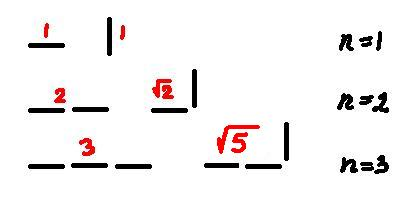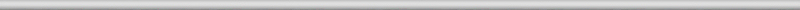## October 27, 2007

### Lengths of digital curves, part 3

Filed under: computer vision/machine vision/AI, reviews, mathematics — Peter @ 8:32 pm

Recall that in the previous posts we discussed what happens if one computes the length of a curve in a digital image as the total sum of distances between consecutive points. The conclusion was that using the length computed this way to evaluate the shapes of objects leads to disastrous results.

What do we do?

Let’s review. Computing lengths of horizontal and vertical segments produces correct results. Computing lengths of diagonal segments leads to a 40% error. To fix that, every time we have a triple of consecutive points arranged in a triangle we should replace 1+1=2 in the computation with √2. The result is that now all 45 degree segments have correct lengths! Great!Great? Not quite. What about 22.5 degree segments? To make matters simpler consider instead segments with 2 horizontal steps followed by 1 vertical. We compute its length as 1+√2, which is about 2.41. Meanwhile the “true” length is √(2^2+1^2) = √5, which is about 2.24. The error is almost 8%!

Once again, what do we do? Very simple, we take into account this new type of segments. Now we have three types: horizontal/vertical, diagonal, and now 2-straight-then-turn. To compute the length of a curve we break it into segments of the three types and add their lengths.

You can predict what happens next. We try 22.5/2 degree – there will still be an error. And so on. There is no exact method to compute the length of a digital curve, locally.

This is the idea – as I understand it – of the paper On Local Definitions of Length of Digital Curves by Mohamed Tajine and Alain Daurat. One breaks a curve into a sequence of “small” (n steps) curves, each small curve is assigned a length (it does not have to be the distance from the beginning to the end), then the length of the original curve is the sum of those. Simple enough. The caveat was discussed previously. As the resolution approaches 0, the length computed this way should converge to the “true” length. Generally, it does not!

The paper proves that this “local” approach can’t produce the exact result no matter how large n is. Of course, you can interpolate the curve and measure the result. But that’s a can of worms that deserves a separate discussion.

The result is interesting. It’s helpful too in the sense that you don’t have to waste your time trying to find a solution to a problem that can’t be solved.

I do have a minor criticism. The curve is a sequence of “small” curves consecutively attached to each other, fine. Once you start to compute the length, however, the way they are attached is thrown out. If you don’t want to lose information, you should allow the curves to overlap, by a single pixel. My guess is that the result would still stand.

Another issue not discussed in the paper is that the error goes down as n increases. This is a good news because it allows one to produce meaningful results in shape evaluation. About that in the next post.